NCERT Exemplar Solutions: Squares & Square Roots & Cubes & Cube Roots

# NCERT Exemplar Solutions: Squares & Square Roots & Cubes & Cube Roots Notes | Study Mathematics (Maths) Class 8 - Class 8

## Document Description: NCERT Exemplar Solutions: Squares & Square Roots & Cubes & Cube Roots for Class 8 2022 is part of Squares & Square Roots for Mathematics (Maths) Class 8 preparation. The notes and questions for NCERT Exemplar Solutions: Squares & Square Roots & Cubes & Cube Roots have been prepared according to the Class 8 exam syllabus. Information about NCERT Exemplar Solutions: Squares & Square Roots & Cubes & Cube Roots covers topics like Exercise Page: 88 and NCERT Exemplar Solutions: Squares & Square Roots & Cubes & Cube Roots Example, for Class 8 2022 Exam. Find important definitions, questions, notes, meanings, examples, exercises and tests below for NCERT Exemplar Solutions: Squares & Square Roots & Cubes & Cube Roots.

Introduction of NCERT Exemplar Solutions: Squares & Square Roots & Cubes & Cube Roots in English is available as part of our Mathematics (Maths) Class 8 for Class 8 & NCERT Exemplar Solutions: Squares & Square Roots & Cubes & Cube Roots in Hindi for Mathematics (Maths) Class 8 course. Download more important topics related with Squares & Square Roots, notes, lectures and mock test series for Class 8 Exam by signing up for free. Class 8: NCERT Exemplar Solutions: Squares & Square Roots & Cubes & Cube Roots Notes | Study Mathematics (Maths) Class 8 - Class 8
 1 Crore+ students have signed up on EduRev. Have you?

Exercise Page: 88

In each of the questions, 1 to 24, write the correct answer from the given four options.
Q.1. 196 is the square of
(a) 11
(b) 12
(c) 14
(d) 16
Ans: (c)
Explanation: Square of 196 is,
= 14 × 14 = 196

Q.2. Which of the following is a square of an even number?
(a) 144
(b) 169
(c) 441
(d) 625
Ans: (a)
Explanation: Square of even number is 144,
= 12 × 12 = 144

Q.3. A number ending in 9 will have the units place of its square as
(a) 3
(b) 9
(c) 1
(d) 6
Ans: (c)
Explanation: Because, the square of a number having 1 or 9 at the units place ends in 1.
Example: – 11 × 11 = 121

Q.4. Which of the following will have 4 at the units place?
(a) 142
(b) 622
(c) 272
(d) 352
Ans: (b)
Explanation: Because, the square of a number having 2 or 8 at the units place ends in 4.
62 × 62 = 3844

Q.5. How many natural numbers lie between 52 and 62?
(a) 9
(b) 10
(c) 11
(d) 12
Ans: (b)
Explanation: The natural numbers lie between 52 and 62 i.e. 25 and 36 are,
26, 27, 28, 29, 30, 31, 32, 33, 34 and 35

Q.6. Which of the following cannot be a perfect square?
(a) 841
(b) 529
(c) 198
(d) All of the above
Ans: (c)
Explanation: Because, we know that the numbers end with 2, 3, 7 and 8 are not perfect squares.

Q.7. The one’s digit of the cube of 23 is
(a) 6
(b) 7
(c) 3
(d) 9
Ans: (b)
Explanation: The one’s digit of cube 23 i.e. 23 × 23 × 23 = 12,167
So, one’s digit is 7

Q.8. A square board has an area of 144 square units. How long is each side of the board?
(a) 11 units
(b) 12 units
(c) 13 units
(d) 14 units
Ans: (b)
Explanation: We know that area of square = Side × Side
= 12 × 12
= 144 square units

Q.9. Which letter best represents the location of √25 on a number line?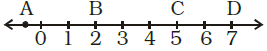(a) A
(b) B
(c) C
(d) D

Ans: (c)
Explanation: √25 = 5 × 5 = 52 = 25

Q.10. If one member of a Pythagorean triplet is 2m, then the other two members are
(a) m, m2 + 1
(b) m2 + 1, m2 – 1
(c) m2, m2 – 1
(d) m2, m + 1
Ans: (b)
Explanation: We know that, for every natural number m > 1, 2m, m2–1 and m2 + 1 form a Pythagorean triplet.

Q.11. The sum of successive odd numbers 1, 3, 5, 7, 9, 11, 13 and 15 is
(a) 81
(b) 64
(c) 49
(d) 36
Ans: (b)
Explanation: = 1 + 3 + 5 + 7 + 9 + 11 + 13 + 15
= 64

Q.12. The sum of first n odd natural numbers is
(a) 2n + 1
(b) n2
(c) n2 – 1
(d) n2 + 1
Ans: (b)
Explanation: The sum of first n odd natural numbers is given by n2.

Q.13. Which of the following numbers is a perfect cube?
(a) 243
(b) 216
(c) 392
(d) 8640
Ans: (b)
Explanation: A perfect cube can always be expressed as the product of triplets of prime factors.
216 = 6 × 6 × 6 = 63

Q.14. The hypotenuse of a right triangle with its legs of lengths 3x × 4x is
(a) 5x
(b) 7x
(c) 16x
(d) 25x
Ans: (a)
Explanation: We know that,
Pythagoras theorem = a = √(b2 + c2)
Where ‘a’ is hypotenuse and b and c are the other two legs of triangle.
Then,
a = √ ((3x)2 + (4x)2)
a = √(9x2 + 16x2)
a = √(9x2 + 16x2)
a = √(9x + 16x)
a = √25x
a = 5x
Hence the hypotenuse of the right triangle is 5x.

Q.15. The next two numbers in the number pattern 1, 4, 9, 16, 25 … are
(a)35, 48
(b) 36, 49
(c) 36, 48
(d) 35,49
Ans: (b)
Explanation: The numbers given in the question are perfect square numbers.
12 = 1, 22 = 4, 32 = 9, 42 = 16, 52 = 25
So, 6 × 6 = 36 and 7 × 7 = 49

Q.16. Which among 432, 672, 522, 592 would end with digit 1?
(a) 432
(b) 672
(c) 522
(d) 592
Ans: (d)
Explanation: Because, the square of a number having 1 or 9 at the units place ends in1.

Q.17. A perfect square can never have the following digit in its ones place.
(a) 1
(b) 8
(c) 0
(d) 6
Ans: (b)

Q.18. Which of the following numbers is not a perfect cube?
(a) 216
(b) 567
(c) 125
(d) 343
Ans: (b)
Explanation: Because, factors of 216 = 6 × 6 × 6
567 = 9 × 9 × 7
125 = 5 × 5 × 5
343 = 7 × 7 × 7

Q.19. 3√1000 is equal to
(a) 10
(b) 100
(c) 1
(d) none of these
Ans: (a)
Explanation: = 3√1000
= 3√(10 × 10 × 10)
= 3√(103)
= 10

Q.20. If m is the square of a natural number n, then n is
(a) the square of m
(b) greater than m
(c) equal to m
(d) √m
Ans: (d)
Explanation: n2 = m
Then,
= n = √m

Q.21. A perfect square number having n digits where n is even will have square root with
(a) n + 1 digit
(b) n/2 digit
(c) n/3 digit
(d) (n + 1)/2 digit
Ans: (b) n/2 digit

Q.22. If m is the cube root of n, then n is
(a) m3
(b) √m
(c) m/3
(d) 3√m
Ans: (a)
Explanation: Given in the question,
3√n = m
n = m3

Q.23. The value of √(248 + √(52 + √144)) is
(a) 14
(b) 12
(c) 16
(d) 13
Ans: (c)
Explanation: √(248 + √(52 + √144))
= √(248 + √(52 + 12))
= √(248 + √(64))
= √(248 + 8)
= √(256)
= 16

Q.24. Given that √4096 = 64, the value of √4096 + √40.96 is
(a) 74
(b) 60.4
(c) 64.4
(d) 70.4
Ans: (d)
Explanation: √4096 + √40.96
= 64 + 6.4
= 70.4

In questions 25 to 48, fill in the blanks to make the statements true.
Q.25. There are _________ perfect squares between 1 and 100.
Solution: There are 8 perfect squares between 1 and 100.
2 × 2 = 4
3 × 3 = 9
4 × 4 = 16
5 × 5 = 25
6 × 6 = 36
7 × 7 = 49
8 × 8 = 64
9 × 9 = 81

Q.26. There are _________ perfect cubes between 1 and 1000.
Solution: There are 8 perfect cubes between 1 and 1000.
2 × 2 × 2 = 8
3 × 3 × 3 = 27
4 × 4 × 4 = 64
5 × 5 × 5 = 125
6 × 6 × 6 = 216
7 × 7 × 7 = 343
8 × 8 × 8 = 512
9 × 9 × 9 = 729

Q.27. The units digit in the square of 1294 is _________.
Solution: The units digit in the square of 1294 is 6.
We know that, 4 or 6 at the units place ends in 6.

Q.28. The square of 500 will have _________ zeroes.
Solution: The square of 500 will have 4 zeroes.
5002 = 500 × 500 = 25000

Q.29. There are _________ natural numbers between n2 and (n + 1)2.
Solution: There are 2n natural numbers between n2 and (n + 1)2.

Q.30. The square root of 24025 will have _________ digits.
Solution: The square root of 24025 will have 3 digits.
The number given in the question has 5 digits,
i.e. n = 5
Then,
= (n + 1)/2
= (5 + 1)/2
= 6/2
= 3

Q.31. The square of 5.5 is _________.
Solution: The square of 5.5 is 30.25.
= 5.52 = 5.5 × 5.5 = 30.25

Q.32. The square root of 5.3 × 5.3 is _________.
Solution: The square root of 5.3 × 5.3 is 5.3.
= √(5.3 × 5.3)
= √(28.09)
= 5.3

Q.33. The cube of 100 will have _________ zeroes.
Solution: The cube of 100 will have 6 zeroes.
= 1003
= 100 × 100 × 100
= 1000000

Q.34. 1m2 = _________ cm2.
Solution: We know that, 1m = 100cm
Then,
1m2 = 10000 cm2

Q.35. 1m3 = _________ cm3.
Solution: We know that, 1m = 100cm
Then,
1m3 = 1000000 cm3

Q.36. Ones digit in the cube of 38 is _________.
Solution: Ones digit in the cube of 38 is 2.
We know that,
38 × 38 × 38 = 54,872

Q.37. The square of 0.7 is _________.
Solution: The square of 0.7 is 0.49.
= 0.72
= 0.7 × 0.7
= 0.49

Q.38. The sum of first six odd natural numbers is _________.
Solution: The sum of first six odd natural numbers is 36.
The first six odd numbers are 1, 3, 5, 7, 9, and 11
Then,
Sum of first six odd numbers = 1 + 3 + 5 + 7 + 9 + 11
= 36

Q.39. The digit at the ones place of 572 is _________.
Solution: The digit at the ones place of 572 is 9.
We know that, 3 or 7 at the units place ends in 9.
= 572
= 57 × 57
= 3249

Q.40. The sides of a right triangle whose hypotenuse is 17cm are _________ and _________.
Solution: For every natural number m > 1, 2m, m2 –1 and m2 + 1 form a Pythagorean triplet.
Now,
m2 + 1 = (2m)2 + (m2 – 1)2
Where,
m2 + 1 = 17
m2 = 17 – 1
m2 = 16
m = √16
m = 4
Then,
2m = 2 × 4
= 8
And,
m– 1 = 4– 1
= 16 -1
= 15

Q.41. √(1.96) = _________.
Solution: We have,
= √(1.96)
= √(196/100)
= √((14 × 14)/(10 × 10))
= √(142 / 102)
= 14/10
= 1.4

Q.42. (1.2)3 = _________.
Solution: (1.2)3 = 12/10
= (12/10) × (12/10) × (12/10)
= 1728/1000
= 1.728

Q.43. The cube of an odd number is always an _________ number.
Solution: The cube of an odd number is always an odd.

Q.44. The cube root of a number x is denoted by _________.
Solution: The cube root of a number x is denoted by 3√x or x1/3

Q.45. The least number by which 125 be multiplied to make it a perfect square is _____________.
Solution: The least number by which 125 be multiplied to make it a perfect square is 5.
125 = 5 × 5 × 5

Q.46. The least number by which 72 be multiplied to make it a perfect cube is _____________.
Solution: The least number by which 72 be multiplied to make it a perfect cube is 3.
72 = 2 × 2 × 2 × 3 × 3

Q.47. The least number by which 72 be divided to make it a perfect cube is _____________.
Solution: The least number by which 72 be divided to make it a perfect cube is 9.

Q.48. Cube of a number ending in 7 will end in the digit _______________.
Solution: Cube of a number ending in 7 will end in the digit 3.

In questions 49 to 86, state whether the statements are true (T) or false (F).
Q.49. The square of 86 will have 6 at the units place.
Solution: True.
The square of a number having 4 or 6 at the units place ends in 6.

Q.50. The sum of two perfect squares is a perfect square.
Solution: False.
Let us take two perfect square 25 and 49.
Then, sum of the two perfect square = 25 + 49 = 74
So, 74 is not perfect square.

Q.51. The product of two perfect squares is a perfect square.
Solution: True.
Let us take two perfect square 4 and 9.
Then, product of two perfect square is = 4 × 9 = 36

Q.52. There is no square number between 50 and 60.
Solution: True.

Q.53. The square root of 1521 is 31.
Solution: False.
The square root of 1521 is 39.

Q.54. Each prime factor appears 3 times in its cube.
Solution: True.
We know that, a perfect cube can always be expressed as the product of triplets of prime factors.

Q.55. The square of 2.8 is 78.4.
Solution: False.
= 2.82
= (28/10)2
= 784/100
= 7.84

Q.56. The cube of 0.4 is 0.064.
Solution: True.
= 0.43
= (4/10)3
= 64/1000
= 0.064

Q.57. The square root of 0.9 is 0.3.
Solution: False.
= √0.9
= 0.9487
0.9 = 0.32
= 0.3 × 0.3
= 0.09

Q.58. The square of every natural number is always greater than the number itself.
Solution: False.
As 1 is a natural number and the square of 1 is not greater itself rather its equal to the number itself
Thus, the given statement is not always true.

Q.59. The cube root of 8000 is 200.
Solution: False
3√8000 = 20

Q.60. There are five perfect cubes between 1 and 100.
Solution: False.
There are only 3 perfect cubes between 1 and 100.
2 × 2 × 2 = 8
3 × 3 × 3 = 27
4 × 4 × 4 = 64

Q.61. There are 200 natural numbers between 1002 and 1012.
Solution: True.
We know that, natural numbers between a and b = b –a – 1
= 1012 – 1002 – 1
= (101 + 100) (101 – 100) – 1

= 201 – 1
= 200

Q.62. The sum of first n odd natural numbers is n2.
Solution: True.
The sum of odd numbers = ∑ (2n – 1)
= (2 × n × (n + 1))/2 –n
= n2 + n – n
= n2

Q.63. 1000 is a perfect square.
Solution: False.
It is not a perfect square.
1000 is a perfect cube, i.e. 10 × 10 × 10 = 1000

Q.64. A perfect square can have 8 as its unit’s digit.
Solution: False.
A perfect square can never have 8 as its unit’s digit.

Q.65. For every natural number m, (2m –1, 2m2 –2m, 2m2 –2m + 1) is a Pythagorean triplet.
Solution: False.
For every natural number m > 1, 2m, m2–1 and m2 + 1 form a Pythagorean triplet.

Q.66. All numbers of a Pythagorean triplet are odd.
Solution: False.
Three natural numbers a, b, c are said to form a Pythagorean triplet if a2 + b2 = c2.
Then,
Pythagorean triplet as, 72 = 62 + 52
6 is not an odd number.

Q.67. For an integer a, a3 is always greater than a2.
Solution: False.
Let us take one negative integer, i.e. -2
Then, -23 = -2 × -2 × -2 = -8
-22 = -2 × -2 = 4
Here, 4 > -8
Hence, -22 is greater than -23.

Q.68. If x and y are integers such that x2 > y2, then x3 > y3.
Solution: False.
Let us take negative integers -2 and -3
Then,
= (-22) > (-12)
= (4) > (1)
and = -23 < -13
= -8 < -1

Q.69. Let x and y be natural numbers. If x divides y, then x3 divides y3.
Solution: True.
If x divides y = y/x is a natural number
and then x3 divides y3 = y3/x3 is a natural number

Q.70. If a2 ends in 5, then a3 ends in 25.
Solution: False.
Let a2 be 152
So, 152 = 225
Then, 153 = 3375

Q.71. If a2 ends in 9, then a3 ends in 7.
Solution: False.
Let a2 be 72
So, 72 = 49
Then, 73 = 343

Q.72. The square root of a perfect square of n digits will have (n + 1)/2 digits, if n is odd.
Solution: True.
Let au assume the perfect square has 9 digits, then its square root has 5 digits
i.e. (9 + 1)/2 = 10/2 = 5

Q.73. Square root of a number x is denoted by √x.
Solution: True.

Q.74. A number having 7 at its ones place will have 3 at the units place of its square.
Solution: False.
Let us take the number having 7 at its ones place i.e.47 and
Its square 472 = 2209

Q.75. A number having 7 at its ones place will have 3 at the ones place of its cube.
Solution: True.
Let us take the number having 7 at its ones place i.e. 37 and
Its square 373 = 50,653
Another number is 273 = 19,683

Q.76. The cube of a one digit number cannot be a two digit number.
Solution: False.
The cube of 3 .i.e. 43 = 4 × 4 × 4 = 64

Q.77. Cube of an even number is odd.
Solution: False.
The cube of an even number is always an even number.
23 = 8
43 = 64

Q.78. Cube of an odd number is even.
Solution: False.
The cube of odd number is always an odd number.
33 = 27
53 = 125

Q.79. Cube of an even number is even.
Solution: True.
The cube of an even number is always an even number.
83 = 512
123 = 1728

Q.80. Cube of an odd number is odd.
Solution: True.
The cube of odd number is always an odd number.
73 = 343

Q.81. 999 is a perfect cube.
Solution: False.

Q.82. 363 × 81 is a perfect cube.
Solution: False.
So, factors of 363 × 81 are 3 × 11 × 11 × 3 × 3 × 3 × 3
= 33 × 11 × 11 × 3 × 3

Q.83. Cube roots of 8 are +2 and –2.
Solution: False.
Because, cube root of 8 is 2 only.
i.e. 2 × 2 × 2 = 8

Q.84. 3√ (8 + 27) = 3√ (8) + 3√ (27).
Solution: False.
Let us consider LHS = 3√ (8 + 27)
= 3√ (35)
Then, RHS = 3√8 + 3√27
= 2 + 3
= 5
By comparing LHS and RHS
LHS ≠ RHS
3√ (35) ≠ 5

Q.85. There is no cube root of a negative integer.
Solution: False.
Let us take -27
Cube root of -27 i.e. 3√-27 = 3√((-3) × (-3) × (-3))
= -3

Q.86. Square of a number is positive, so the cube of that number will also be positive.
Solution: False.
Let us take -3
Now, square of the -3 i.e. -32 = 9
Then, cube of the same number i.e. -33 = -27

Solve the following questions.
Q.87. Write the first five square numbers.
Solution: The first five square numbers are,
1 × 1 = 1
2 × 2 = 4
3 × 3 = 9
4 × 4 = 16
5 × 5 = 25

Q.88. Write cubes of first three multiples of 3.
Solution: The first three multiple of 3 are 3, 6 and 9
Then,
Cubes of first three multiples of 3,
= 33 = 3 × 3 × 3 = 27
= 63 = 6 × 6 × 6 = 216
= 93 = 9 × 9 × 9 = 729

Q.89. Show that 500 is not a perfect square.
Solution: Factors of 500 are 2 × 2 × 5 × 5 × 5
Now,
Group the factors into pairs,
500 = (2 × 2) × (5 × 5 × 5)
= 22 × 53
So, the prime factors 2 and 5 do not occur in pairs.
Therefore, 500 is not a perfect square.

Q.90. Express 81 as the sum of first nine consecutive odd numbers.
Solution: The first nine consecutive odd numbers are 1, 3, 5, 7, 9, 11, 13, 15 and 17
Then,
We know that 81 is a perfect square,
81 = (9)2
Now,
The sum of first nine consecutive odd numbers,
= 1 + 3 + 5 + 7 + 9 + 11 + 13 + 15 + 17
= 81

Q.91. Using prime factorisation, find which of the following are perfect squares.
(a) 484
Solution:
First we have to find out the factors by using prime factorisation method.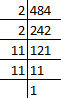So, prime factors of 484 = 2 × 2 × 11 × 11
Now, grouping the prime factors = (2 × 2) × (11 × 11)
= 22 × 112
There is no unpaired factor remains here.
∴484 is a perfect square.

(b) 11250
Solution: First we have to find out the factors by using prime factorisation method.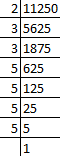So, prime factors of 11250 = 2 × 3 × 3 × 5 × 5 × 5 × 5
Now, grouping the prime factors = 2 × (3 × 3) × (5 × 5) × (5 × 5)
= 2 × 32 × 52 × 52
Factor 2 has no pair.
∴ 11250 is not a perfect square.

(c) 841
Solution: First we have to find out the factors by using prime factorisation method.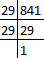So, prime factors of 841 = 29 × 29
Now, grouping the prime factors = (29 × 29)
= 292
There is no unpaired factor remains here.
∴ 841 is a perfect square.

(d) 729
Solution: First we have to find out the factors by using prime factorisation method.So, prime factors of 729 = 3 × 3 × 3 × 3 × 3 × 3
Now, grouping the prime factors = (3 × 3) × (3 × 3) × (3 × 3)
= 32 × 3× 32
There is no unpaired factor remains here.
∴ 729 is a perfect square.

Q.92. Using prime factorisation, find which of the following are perfect cubes.
(a)128
Solution: First we have to find out the factors by using prime factorisation method.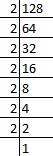So, prime factors of 128 = 2 × 2 × 2 × 2 × 2 × 2 × 2
Now, grouping the prime factors = (2 × 2 × 2) × (2 × 2 × 2) × 2
= 23 × 2× 2
Factor 2 remains unpaired after grouping in triplets.
∴128 is not a perfect cube.

(b) 343
Solution: First we have to find out the factors by using prime factorisation method.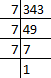So, prime factors of 343 = 7 × 7 × 7
Now, grouping the prime factors = (7 × 7 × 7)
= 73
There is no unpaired factor remains here.
∴ 343 is a perfect cube.

(c) 729
Solution: First we have to find out the factors by using prime factorisation method.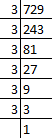So, prime factors of 729 = 3 × 3 × 3 × 3 × 3 × 3
Now, grouping the prime factors = (3 × 3 × 3) × (3 × 3 × 3)
= 33 × 33
There is no unpaired factor remains here.
∴ 729 is a perfect cube.

(d) 1331
Solution: First we have to find out the factors by using prime factorisation method.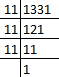So, prime factors of 1331 = 11 × 11 × 11
Now, grouping the prime factors = (11 × 11 × 11)
= 113
There is no unpaired factor remains here.
∴ 1331 is a perfect cube.

Q.93. Using distributive law, find the squares of
(a)101
Solution: By using distributive law,
We have,
101 = 100 + 1
So,
1012 = (100 + 1)2
= (100 + 1) (100 + 1)
= 100 (100 + 1) + 1 (100 + 1)
= ((100 × 100) + (100 × 1)) + ((1 × 100) + (1 × 1))
= 10000 + 100 + 100 + 1
= 10201
∴ The square of the given number i.e. 1012 = 10201

(b) 72
Solution: By using distributive law,
We have,
72 = 70 + 2
So,
722 = (70 + 2)2
= (70 + 2) (70 + 2)
= 70 (70 + 2) + 2 (70 + 2)
= ((70 × 70) + (70 × 2)) + ((2 × 70) + (2 × 2))
= 4900 + 140 + 140 + 4
= 5184
∴ The square of the given number i.e. 722 = 5184

Q.94. Can a right triangle with sides 6cm, 10cm and 8cm be formed? Give reason.
Solution: From the question it is given that,
Sides of the right angle triangle are 6 cm, 10 cm and 8 cm
We know that, the square one side must be equal to the sum of square of other two sides of right angle triangle.
i.e. a2 = b2 + c2
Where, a = 10 cm, b = 8 cm, c = 6 cm
102 = 82 + 62
100 = 64 + 36
100 = 100
∴ Right angle triangle is formed by the given sides 6cm, 10cm and 8cm.

Q.95. Write the Pythagorean triplet whose one of the numbers is 4.
Solution: Given, one of the number in the Pythagorean triplet i.e. 4.
Then,
2m = 4
m = 4/2
m = 2
So,
= m2 + 1
= 2+ 1
= 4 + 1
= 5
And,
= m2 – 1
= 22 – 1
= 4 – 1
= 3
∴ The Pythagorean triplet is 3, 4 and 5.

Q.96. Using prime factorisation, find the square roots of
(a) 11025
Solution: First we have to find out the factors by using prime factorisation method.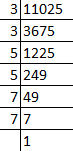So, prime factors of 11025 = 3 × 3 × 5 × 5 × 7 × 7
Now, grouping the prime factors = (3 × 3) × (5 × 5) × (7 × 7)
Then, Square root of 11025 = √11025
= √((3 × 3) × (5 × 5) × (7 × 7))
= √(32 × 52 × 72)
= 3 × 5 × 7
= 105
∴ The Square root of 11025 is 105.

(b) 4761
Solution: First we have to find out the factors by using prime factorisation method.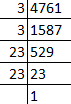So, prime factors of 4761 = 3 × 3 × 23 × 23
Now, grouping the prime factors = (3 × 3) × (23 × 23)
Then, Square root of 4761 = √4761
= √((3 × 3) × (23 × 23))
= √(32 × 232)
= 3 × 23
= 69
∴ The Square root of 4761 is 69.

Q.97. Using prime factorisation, find the cube roots of
(a) 512
Solution: First we have to find out the factors by using prime factorisation method.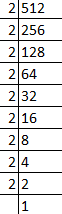So, prime factors of 512 = 2 × 2 × 2 × 2 × 2 × 2 × 2 × 2 × 2
Now, grouping the prime factors = (2 × 2 × 2) × (2 × 2 × 2) × (2 × 2 × 2)
Then, cube root of 512 = 3√512
= 3√((2 × 2 × 2) × (2 × 2 × 2) × (2 × 2 × 2))
= 3√(23 × 23 × 23)
= 2 × 2 × 2
= 8
∴ The cube root of 512 is 8.

(b) 2197
Solution: First we have to find out the factors by using prime factorisation method.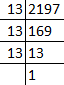So, prime factors of 2197 = 13 × 13 × 13
Now, grouping the prime factors = (13 × 13 × 13)
Then, cube root of 2197 = 3√2197
= 3√(13 × 13 × 13)
= 3√(133)
= 13
∴ The cube root of 2197 is 13.

Q.98. Is 176 a perfect square? If not, find the smallest number by which it should be multiplied to get a perfect square.
Solution: First we have to find out the factors by using prime factorisation method.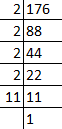So, prime factors of 176 = 2 × 2 × 2 × 2 × 11
Now, grouping the prime factors = (2 × 2) × (2 × 2) × (11)
= 22 × 22 × 11
Factor 11 has no pair.
∴ 176 is not a perfect square.
The smallest number it should be multiplied to get a perfect square is 11.
Then,
176 × 11 = 1936
Factors of 1936 = 2 × 2 × 2 × 2 × 11 × 11
= √1936
= √(22 × 22 × 112)
= 2 × 2 × 11
= 44
∴ Square root of 1936 is 44.

Q.99. Is 9720 a perfect cube? If not, find the smallest number by which it should be divided to get a perfect cube.
Solution: First we have to find out the factors by using prime factorisation method.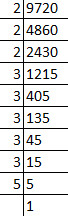So, prime factors of 9720 = 2 × 2 × 2 × 3 × 3 × 3 × 3 × 3 × 5
Now, grouping the prime factors = (2 × 2 × 2) × (3 × 3 × 3) × 3 × 3 × 5
= 23 × 33 × 3 × 3 × 5
Factors 3 and 4 has no pair.
∴ 9720 is not a perfect cube.
The smallest number it should be divided to get a perfect cube is 3 × 3 × 5 = 45.
Then,
= 9720 ÷ 45
= 216
Factors of 216 = (2 × 2 × 2) × (3 × 3 × 3)
3√216 = 3√((2 × 2 × 2) × (3 × 3 × 3))
= 3√(23 × 32)
= 6

Q.100. Write two Pythagorean triplets each having one of the numbers as 5.
Solution: Given, one of the number in the Pythagorean triplet i.e. 5.
= m2 + 1 = 5
= m2 = 4
= m = 2
Then,
2m = 2 × 2
m = 4
So,
And,
= m2 – 1
= 22 – 1
= 4 – 1
= 3
∴ The Pythagorean triplet is 3, 4 and 5.
Similarly,
The other Pythagorean triplet is 5, 12 and 13.

Q.101. By what smallest number should 216 be divided so that the quotient is a perfect square. Also find the square root of the quotient.
Solution: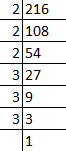Factors of 216 = 2 × 2 × 2 × 3 × 3 × 3
Now, grouping the prime factors = (2 × 2) × 2 × (3 × 3) × 3
Factors 2 and 3 has no pair.
∴ 216 is not a perfect square.
The smallest number it should be divided to get a perfect square is 3 × 2 = 6.
Then,
= 216 ÷ 6
= 36
Factors of 36 = 6 × 6
√36 = √(6× 6)
= √(6)
= 6
∴ 36 is a perfect square and 6 is the square root of 36.

Q.102. By what smallest number should 3600 be multiplied so that the quotient is a perfect cube. Also find the cube root of the quotient.
Solution: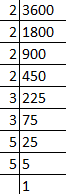Factors of 3600 = 2 × 2 × 2 × 2 × 3 × 3 × 5 × 5
Now, grouping the prime factors = (2 × 2 × 2) × 2 × 3 × 3 × 5 × 5
Factors 2, 3 and 5 has no pair.
∴ 3600 is not a perfect cube.
The smallest number it should be multiplied to get a perfect cube is 2 × 2 × 3 × 5 = 60
Then,
Product = 3600 × 60 = 216000
Factors of 21600 = 2 × 2 × 2 × 2 × 2 × 2 × 3 × 3 × 3 × 5 × 5 × 5
= 3√216000
= 3√(23 × 23 × 33 × 53)
= 2 × 2 × 3 × 5
= 60

Q.103. Find the square root of the following by long division method.
(a) 1369
Solution: We have,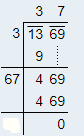∴ √1369 = 37

(b) 5625
Solution: We have,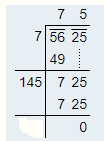∴ √5625 = 75

Q.104. Find the square root of the following by long division method.
(a) 27.04
Solution: We have,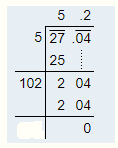∴ √27.04 = 5.2

(b) 1.44
Solution: We have,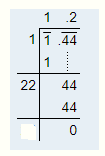∴ √1.44 = 1.2

Q.105. What is the least number that should be subtracted from 1385 to get a perfect square? Also find the square root of the perfect square.
Solution: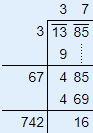From the long division method, the least number that should be subtracted from 1385 to get a perfect square is 16.
Now,
= 1385 – 16
= 1369
So,
√1369 = √(37 × 37)
= √372
= 37
∴ The square root of a perfect square number 1369 is 37.

Q.106. What is the least number that should be added to 6200 to make it a perfect square?
Solution: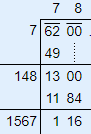So, 782 = 6084
By comparing 6084 and 6200, 6084 < 6200
Then, next perfect square is 792 = 6241
∴ The least number is (6241 – 6200) = 41, which should be added to 6200 to get a perfect square.
The required perfect square number is 6241
So,
√6241 = √(41 × 41)
= √412
= 41

Q.107. Find the least number of four digits that is a perfect square.
Solution: We know that, the least number of four digit is 1000
By using long division method we can able to find a perfect square,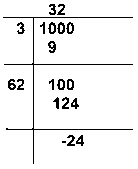∴ The least number of four digits that is a perfect square = 1000 + 24
= 1024

Q.108. Find the greatest number of three digits that is a perfect square.
Solution: We know that, the greatest number of three digit is 999.
By using long division method we can able to find a perfect square,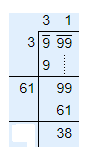∴ The greatest number of three digits that is a perfect square = 999 – 38
= 961

Q.109. Find the least square number which is exactly divisible by 3, 4, 5, 6 and 8.
Solution:
First we have to find out the LCM of 3, 4, 5, 6 and 8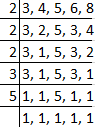So, LCM of 3, 4, 5, 6 and 8 = 2 × 2 × 2 × 3 × 5
= 120
Now, grouping the factors, (2× 2) × 2× 3× 5
i.e. 2,3 and 5 is not able to make their pair.
So, to make it perfect square, 120 must be multiplied with 2× 3× 5 = 30
Then,
= 120× 30 = 3600
∴ 3600 is least square number which is exactly divisible by 3, 4, 5, 6, and 8.

Q.110. Find the length of the side of a square if the length of its diagonal is 10cm.
Solution: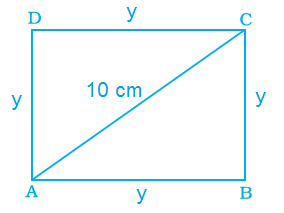From the question it is given that,
Length of Diagonal = 10 cm
Let us assume square ABCD.
Sides AB = y, BC = y, CD = y, DA = y
Diagonal AC = 10 cm
Now, consider triangle ABC
By the rule of Pythagoras theorem,
AC2 = AB2 + BC2
102 = y2 + y2
100 = 2y2
y2 = 100/2
y2 = 50
y = √50 cm
y = 5√2 cm
∴The length of the side of square is √50 cm or 5√2.

Q.111. A decimal number is multiplied by itself. If the product is 51.84, find the number.
Solution: Let us assume decimal number be ‘a’.
We know that, a × a = a2
So,
a × a = 51.84
a2 = 51.84
a = √51.84
The required number is found out by using long division method.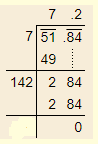Then,
a = √51.84
a = 7.2
∴ 7.2 is the decimal number is multiplied by itself and its product is 51.84.

Q.112. Find the decimal fraction which when multiplied by itself gives 84.64.
Solution: Let us assume decimal fraction be ‘a’.
We know that, a × a = a2
So,
a × a = 84.64
a2 = 84.64
a = √84.64
The required number is found out by using long division method.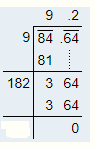Then,
a = √84.64
a = 9.2
∴ 9.2 is the decimal fraction is multiplied by itself and its product is 84.64.

Q.113. A farmer wants to plough his square field of side 150m. How much area will he have to plough?
Solution: From the question it is given that,
Length of the side of square field = 150 m
We know that, are of square = side × side
So,
Area of the square field = 150 × 150
= 22,500 m2
∴ The farmer have to plough 22,500 m2 area.

Q.114. What will be the number of unit squares on each side of a square graph paper if the total number of unit squares is 256?
Solution: From the question it is given that,
The total number of unit squares is = 256.
Let us assume the number be ‘a’
We know that, a × a = a2
So,
a × a = 256
a2 = 256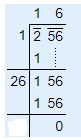a = √256
a = √(16 × 16)
a = √162
a = 16
∴ The number of unit squares are 16.

Q.115. If one side of a cube is 15m in length, find its volume.
Solution: From the question it is given that,
Length of one side of the a cube = 15 m
We know that, volume of cube = (side)3
= 153
= 15 × 15 × 15
= 3375 m3
∴ The volume of cube is 3375 m3.

Q.116. The dimensions of a rectangular field are 80m and 18m. Find the length of its diagonal.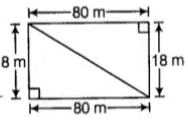Solution: From the question it is given that,
Length of rectangular field = 80 m
Breadth of rectangular field = 18 m
Then,
Length of diagonal = √(length2 + breadth2)
= √(802 + 182)
= √(6400 + 324)
= √6724
= 82 m
∴ The Length of diagonal is 82 m.

Q.117. Find the area of a square field if its perimeter is 96m.
Solution: Given, perimeter of the square field = 96 m
We know that, perimeter of square = 4 × side
Then,
96 = 4 × side
Side = 96/4
Side = 24 m
So, the length of the side of square = 24 m
Now,
Area of the square field = (side)2
= 242
= 576 m2
∴ The area of the square field is 576m2.

Q.118. Find the length of each side of a cube if its volume is 512 cm3.
Solution: From the question it is given that,
Volume of the cube = 512 cm3
We know that,
Volume of cube = side3
512 = side3

By taking cube root on both the side,
3√512 = side
Side = 3√(8 × 8 × 8)
Side = 3√ (8)3
Side = 8 cm
∴ The length of each side of a cube is 8 cm.

Q.119. Three numbers are in the ratio 1:2:3 and the sum of their cubes is 4500. Find the numbers.
Solution: Let us assume the three number be a, 2a, 3a
Then,
Given, sum of cube of three numbers is 4500
i.e. a3 + (2a)3 + (3a)3 = 4500
a3 + 8a3 + 27a3 = 4500
36a3 = 4500
a3 = 4500/36
a3 = 125
a = 3√125
a = 3√(5 × 5 × 5)
a = 3√(53)
a = 5
∴ The numbers are, a = 5
2a = 2 × 5 = 10
3a = 3 × 5 = 15

Q.120. How many square metres of carpet will be required for a square room of side 6.5m to be carpeted.
Solution: Given, side of square room = 6.5 m
So, area of square room = 6.52
= 6.5 × 6.5
= 42.25 m2

Q.121. Find the side of a square whose area is equal to the area of a rectangle with sides 6.4m and 2.5m.
Solution: From the question it is given that,
Length of rectangle = 6.4 m
Breadth of rectangle = 2.5 m
Area of rectangle = length × breadth
= 6.4 × 2.5
= 16 m2
And also it’s given in the question i.e. area of square is equal to the area of rectangle.
Let us assume area of square be ‘a’.
Area of square = Area of rectangle
a × a = 16
a2 = 16
By taking square root on both side
a = √16
a = √(4 × 4)
a = √42
a = 4m2
We know that, area of square = side2
4 = side2
By taking square root on both side
side = √4
side = √(2 × 2)
side = √22
side = 2m

Q.122. Difference of two perfect cubes is 189. If the cube root of the smaller of the two numbers is 3, find the cube root of the larger number.
Solution: From the question it is given that,
Difference of two perfect cubes = 189
The cube root of the smaller of the two numbers = 3
So, cube of smaller number = 33
= 3 × 3 × 3
= 27
Let us assume the cube root of larger number be a3
Then,
As per the condition given in the question,
a3 – 27 = 189
a3 = 189 + 27
a3 =216
By taking cube root on both side,
a = 3√216
a = 3√(6 × 6 × 6)
a = 3√(63)
a = 6
∴ The cube root of the larger number is 6.

Q.123. Find the number of plants in each row if 1024 plants are arranged so that number of plants in a row is the same as the number of rows.
Solution: From the question it is given that total number of plants arranged = 1024
Let us assume number of plants in each row be ‘a’
As per the condition given in the question,
Number of plants in a row is the same as the number of rows i.e. ‘a’
Then,
Total number of plants = a × a
1024 = a2
By taking square root on both side,
a = √(1024)
a = √(32 × 32)
a = √(32)2
a = 32
∴ The number of plants in each row is 32.

Q.124. A hall has a capacity of 2704 seats. If the number of rows is equal to the number of seats in each row, then find the number of seats in each row.
Solution: From the question it is given that total number of seats = 2704
Let us assume number of seats in each row be ‘a’
As per the condition given in the question,
Number of rows is equal to the number of seats in each row i.e. ‘a’
Then,
Total number of seats = a × a
2704 = a2
By taking square root on both side,
a = √(2704)
a = √(52 × 52)
a = √(52)2
a = 52
∴ The number of seats in each row is 52.

Q.125. A General wishes to draw up his 7500 soldiers in the form of a square. After arranging, he found out that some of them are left out. How many soldiers were left out?
Solution: From the question it is given that total number of soldiers = 7500
By using long division method we can able to find number of soldiers left out,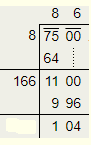∴ 104 soldiers were left out.

Q.126. 8649 students were sitting in a lecture room in such a manner that there were as many students in the row as there were rows in the lecture room. How many students were there in each row of the lecture room?
Solution: From the question it is given that,
Total number of students were sitting in a lecture room = 8649
Let us assume number of students in each row be ‘a’
Then,
Total number of students = a × a
8649 = a2
By taking square root on both side,
a = √(8649)
a = √(93 × 93)
a = √(93)2
a = 93
∴ The number of students in each row is 93.

Q.127. Rahul walks 12m north from his house and turns west to walk 35m to reach his friend’s house. While returning, he walks diagonally from his friend’s house to reach back to his house. What distance did he walk while returning?
Solution: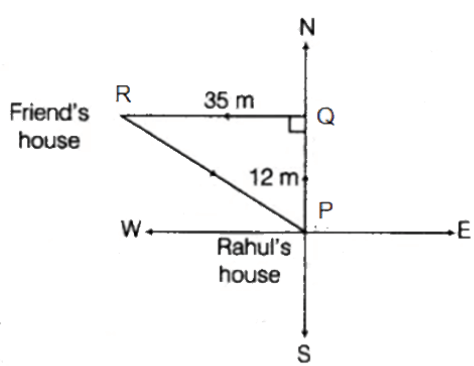From above the figure,
Consider the triangle PQR.
We know that, Pythagoras theorem,
PR2 = PQ2 + QR2
PR2 = 122 + 352
PR2 = 144 + 1225
PR2 = 1369
PR = √1369
PR = 37m
∴ Rahul walked 37m distance for returning to his house.

Q.128. A 5.5m long ladder is leaned against a wall. The ladder reaches the wall to a height of 4.4m. Find the distance between the wall and the foot of the ladder.
Solution:
Let us consider the right angle triangle PQR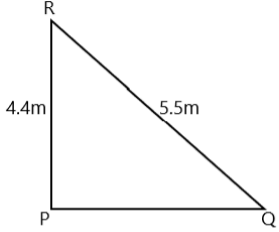Given,
Long ladder i.e. RQ = 5.5 m
The ladder reaches the wall to a height i.e. PR = 4.4 m
From Pythagoras theorem,
RQ2 = RP2 + PQ2
5.52 = 4.42 + PQ2
PQ2 = 5.52 – 4.42
PQ = √(5.52 – 4.42)
PQ = √(30.25 – 19.36)
PQ = √10.89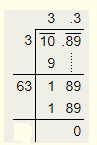PQ = 3.3
∴ The distance between the wall and the foot of the ladder is 3.3 m.

Q.129. A king wanted to reward his advisor, a wise man of the kingdom. So he asked the wiseman to name his own reward. The wiseman thanked the king but said that he would ask only for some gold coins each day for a month. The coins were to be counted out in a pattern of one coin for the first day, 3 coins for the second day, 5 coins for the third day and so on for 30 days. Without making calculations, find how many coins will the advisor get in that month?
Solution: From the question it is given that,
Total coins the advisor get at the end = 1 + 3 + 5 +…..
The coins order is in the series of odd natural number,
So, the number of terms (n) = 30
Then,
Sum of odd natural numbers = n2
= 302
= 900
∴ Total coins the advisor get at the end is 900.

Q.130. Find three numbers in the ratio 2:3:5, the sum of whose squares is 608.
Solution: Let us assume the three number be 2a, 3a, 5a
Then,
Given, sum of squares of three numbers is 608
i.e. (2a)2 + (3a)2 + (5a)2 = 608
4a2 + 9a2 + 25a2 = 608
38a2 = 608
a2 = 608/38
a2 = 16
a = √16
a = √(4 × 4)
a = √(42)
a = 4
∴ The numbers are, 2a = 2 × 4 = 8
3a = 3 × 4 = 12
5a = 5× 4 = 20

Q.131. Find the smallest square number divisible by each one of the numbers 8, 9 and 10.
Solution:
First we have to find out the LCM of 8, 9 and 10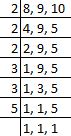So, LCM of 8, 9, and 10 = 2 × 2 × 2 × 3 × 3 × 5
= 360
Now, grouping the factors, (2× 2) × 2 × (3 × 3) × 5
i.e. 2 and 5 is not able to make their pair.
So, to make it perfect square, 360 must be multiplied with 2 × 5 = 10
Then,
= 360 × 10 = 3600
∴ 3600 is least square number which is exactly divisible by 8, 9, and 10.

Q.132. The area of a square plot is 101 1/400 m2. Find the length of one side of the plot.
Solution: From the question it is given that,
Area of a square plot =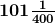m2 = 40401/400
We know that, area of square plot = side2
(40401/400) = side2
By taking square root on both side,
Side = √(40401/400)
= √((201 × 201)/(20 × 20))
= √(2012/202)
= 201/20
=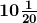m
∴ the length of one side of the plot ism

Q.133. Find the square root of 324 by the method of repeated subtraction.
Solution: From the question it is given that 324
Now, we subtract successive odd numbers starting from 1 as:
324 – 1 = 323
323 – 3 = 320
320 – 5 = 315
315 – 7 = 308
308 – 9 = 299
299 – 11 = 288
288 – 13 = 275
275 – 15 = 260
260 – 17 = 243
243 – 19 = 224
224 – 21 = 203
203 – 23 = 180
180 – 25 = 155
155 – 27 = 128
128 – 29 = 99
99 – 31 = 68
68 – 33 = 35
35 – 35 = 0
Here we see 324 reduces to 0 after subtracting 18 odd numbers.
Therefore the square root of 324 is 18.

Q.134. Three numbers are in the ratio 2:3:4. The sum of their cubes is 0.334125. Find the numbers.
Solution: Let us assume the three number be 2a, 3a, 4a
Then,
Given, sum of cube of three numbers is 0.334125
i.e. (2a)3 + (3a)3 + (4a)3 = 0.334125
8a3 + 27a3 + 64a3 = 0.334125
99a3 = 0.334125
a3 = 0.334125/99
a3 = 0.003375
a3 = 3375/1000000
a = 3√(3375/1000000)
a = 3√((15 × 15 × 15)/(10 × 10 × 10 × 10 × 10 × 10))
a = 3√(153/106)
a = 15/1000
a = 0.015
∴ The numbers are, 2a = 2 × 0.015 = 0.03
3a = 3 × 0.015 = 0.045
4a = 4 × 0.015 = 0.06

Q.135. Evaluate: 3√27 + 3√0.008 + 3√0.064
Solution: = 3√27 + 3√0.008 + 3√0.064
= 3√(3 × 3 × 3) + (3√(8/1000)) + 3√(64/1000)
= 3√(33) + (3√(2 × 2 × 2)/(10 × 10 × 10)) + 3√((4 × 4 × 4)/(10 × 10 × 10))
= 3 + (3√(23/103) + 3√(43/103)
= 3 + (2/10) + (4/10)
= 3 + 0.2 + 0.4
= 3.6

Q.136. {(52 + (122)1/2)}3
Solution: Given, {(52 + (122)1/2)}3
= {(25 + (144)1/2)}3
= {(25 + (12)}3
= {37}3
= 37 × 37 × 37
= 50,653

Q.137. {(62 + (82)1/2)}3
Solution: Given, {(62 + (82)1/2)}3
= {(36 + (64)1/2)}3
= {(36 + (8)}3
= {44}3
= 44 × 44 × 44
= 85,184

Q.138. A perfect square number has four digis, none of which is zero. The digits from left to right have values that are: even, even, odd, even. Find the number.
Solution: Let us assume PQRS is perfect square,
Where, P = even, Q = even, R = odd, S = even
So, 8836 is the perfect square.

Q.139. Put three different numbers in the circles so that when you add the numbers at the end of each line you always get a perfect square.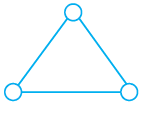Solution: 6, 19 and 30 are the three numbers in which, when we add the end of each line we always get a perfect square.
6 + 19 = 25
6 + 30 = 36
19 + 30 = 49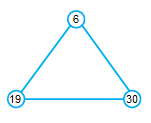Q.140. The perimeters of two squares are 40 and 96 metres respectively. Find the perimeter of another square equal in area to the sum of the first two squares.
Solution: From the question it is given that,
Perimeters of first squares = 40m
Perimeter of second square = 96m
Let us assume side of first square = x1
and assume side of second square = x2
WKT, perimeter of first square = 4 × side
40 = 4x1
X1 = 40/4
X1 = 10 m
Then,
Perimeter of second square = 4 × side
96 = 4x2
X2 = 96/4
X2 = 24 m
As per the condition given in the question area of another square is equal to the first two squares.
Let us assume y2 be the area of another square.
So,
y2 = x12 + x22
y2 = 102 + 242
y2 = 100 + 576
y = √(676)
y = 26 m
Then,
Perimeter of another square = 4 × side
= 4 × y
= 4 × 26
= 104 m

Q.141. A three digit perfect square is such that if it is viewed upside down, the number seen is also a perfect square. What is the number?
(Hint: The digits 1, 0 and 8 stay the same when viewed upside down, whereas 9 becomes 6 and 6 becomes 9.)
Solution: A three digit perfect square is such that if it is viewed upside down are 196 and 961.

Q.142. 13 and 31 is a strange pair of numbers such that their squares 169 and 961 are also mirror images of each other. Can you find two other such pairs?
Solution: First pair is 12 and 21
Squares of numbers, 122 = 144 and 212 = 441
Second pair is 102 and 201
Squares of numbers, 1022 = 10404 and 2012 = 40401

The document NCERT Exemplar Solutions: Squares & Square Roots & Cubes & Cube Roots Notes | Study Mathematics (Maths) Class 8 - Class 8 is a part of the Class 8 Course Mathematics (Maths) Class 8.
All you need of Class 8 at this link: Class 8

## Mathematics (Maths) Class 8

187 videos|321 docs|48 tests
 Use Code STAYHOME200 and get INR 200 additional OFF

## Mathematics (Maths) Class 8

187 videos|321 docs|48 tests

Track your progress, build streaks, highlight & save important lessons and more!

,

,

,

,

,

,

,

,

,

,

,

,

,

,

,

,

,

,

,

,

,

;